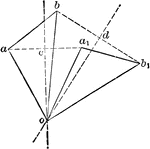### Periodic Center of Motion

Illustration showing the periodic center of motion as it often happens when two positions of a line…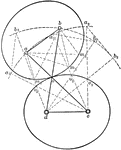### Centroid

Illustration showing a centroid, "the curve passing through the successive positions of the instantaneous…### Motion of Open Curves

"Since these curves are not closed, one pair cannot be used for continuous motion; but a pair of such…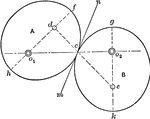### Rolling of Equal Ellipses

"If two equal ellipses, each turning about one of its foci, are placed in contact in such a way that…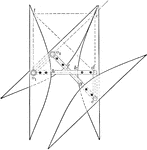### Rolling of Equal Hyperbolas

Illustration of the rolling of equal hyperbolas. If two equal hyperbolas are placed so that the distances…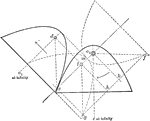### Rolling of Equal Parabolas

Illustration of the rolling of equal parabolas. "The two parabolas may be considered as two ellipses…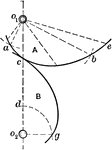### Construction of Logarithmic Spiral

Illustration showing how a rolling logarithmic spiral can be constructed by taking successive values…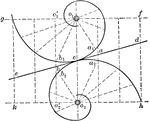### Rolling of Logarithmic Spirals

Illustration showing the rolling of two logarithmic spirals of equal obliquity.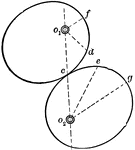### Rolling of Non-cylindrical Surfaces

Illustration showing that the rolling of non-cylindrical surfaces. "If the angular velocity ratio of…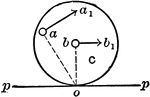### Section of Rolling Surfaces

Illustration showing a section of the rolling surfaces by a plane perpendicular to their straight line…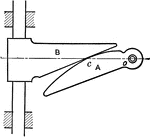### Logarithmic Spiral Sector

In this illustration, "the driven surface of the slide coincides with the tangent to the spiral, the…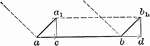### Motion of Translation

Illustration showing the motion of translation of two parallel motions.